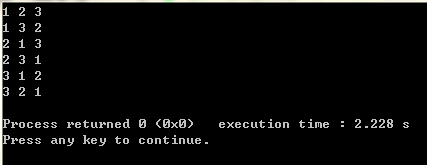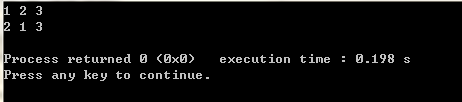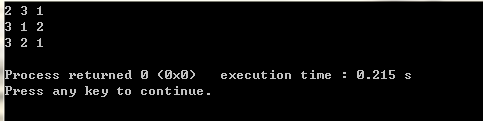# C++中全排列函数next_permutation 用法

http://blog.sina.com.cn/s/blog_9f7ea4390101101u.html

http://blog.csdn.net/ac_gibson/article/details/45308645

next_permutation函数

组合数学中经常用到排列，这里介绍一个计算序列全排列的函数：next_permutation（start,end），和prev_permutation（start,end）。这两个函数作用是一样的，区别就在于前者求的是当前排列的下一个排列，后一个求的是当前排列的上一个排列。至于这里的“前一个”和“后一个”，我们可以把它理解为序列的字典序的前后，严格来讲，就是对于当前序列pn，他的下一个序列pn+1满足：不存在另外的序列pm，使pn<pm<pn+1.

#include <algorithm>

bool next_permutation(iterator start,iterator end)

#include <iostream>
#include <algorithm>
using namespace std;
int main()
{
int num={1,2,3};
do
{
cout<<num<<" "<<num<<" "<<num<<endl;
}while(next_permutation(num,num+3));
return 0;
}next_permutation 自定义比较函数 POJ 1256

//'A'<'a'<'B'<'b'<...<'Z'<'z'.
#include<iostream> //poj 1256 Anagram
#include<string>
#include<algorithm>
using namespace std;
int cmp(char a,char b)
{
if(tolower(a)!=tolower(b))//tolower 是将大写字母转化为小写字母.
else
return a<b;
}
int main()
{
char ch;
int n;
cin>>n;
while(n--)
{
scanf("%s",ch);
sort(ch,ch+strlen(ch),cmp);
do
{
printf("%s\n",ch);
}while(next_permutation(ch,ch+strlen(ch),cmp));
}
return 0;
}

©️2019 CSDN 皮肤主题: 编程工作室 设计师: CSDN官方博客